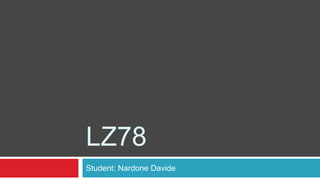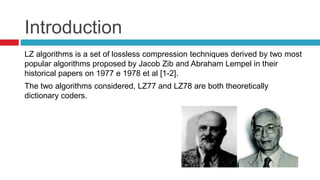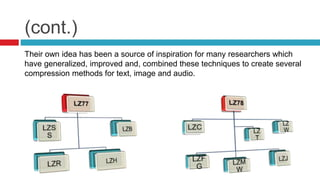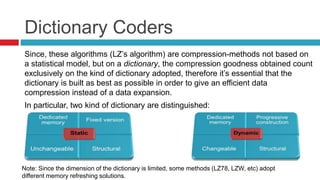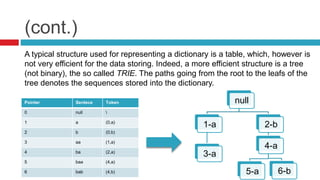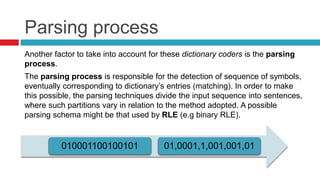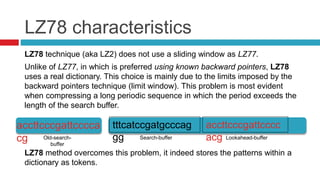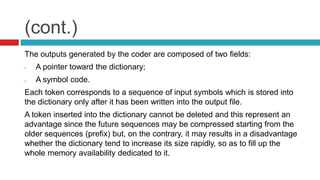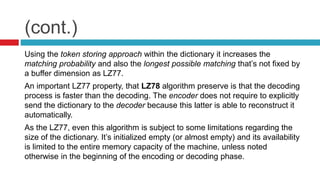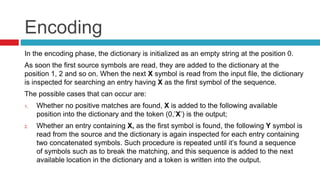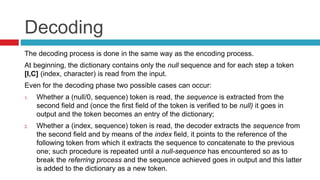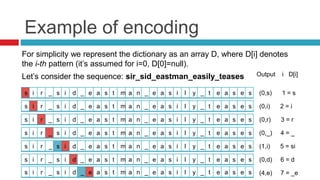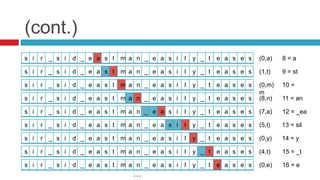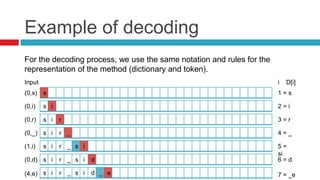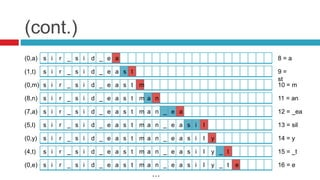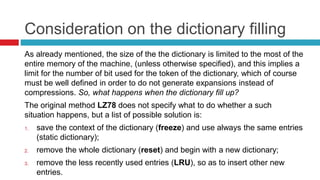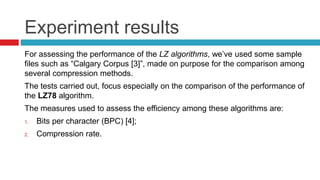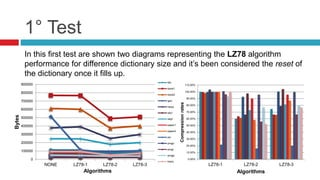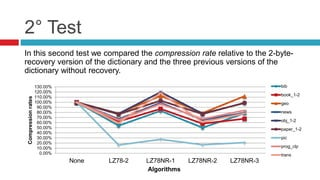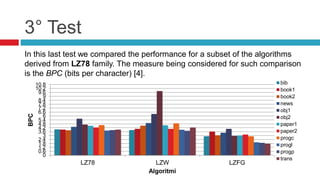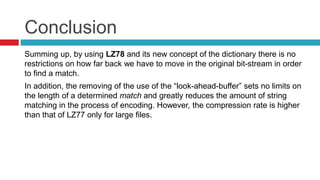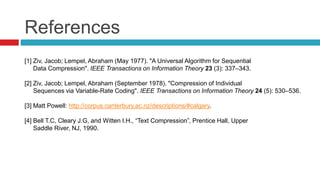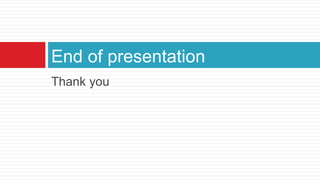1 of 23

### LZ78

1. LZ78 Student: Nardone Davide
2. Introduction LZ algorithms is a set of lossless compression techniques derived by two most popular algorithms proposed by Jacob Zib and Abraham Lempel in their historical papers on 1977 e 1978 et al [1-2]. The two algorithms considered, LZ77 and LZ78 are both theoretically dictionary coders.
3. (cont.) Their own idea has been a source of inspiration for many researchers which have generalized, improved and, combined these techniques to create several compression methods for text, image and audio.
4. Dictionary Coders Since, these algorithms (LZ’s algorithm) are compression-methods not based on a statistical model, but on a dictionary, the compression goodness obtained count exclusively on the kind of dictionary adopted, therefore it’s essential that the dictionary is built as best as possible in order to give an efficient data compression instead of a data expansion. In particular, two kind of dictionary are distinguished: Note: Since the dimension of the dictionary is limited, some methods (LZ78, LZW, etc) adopt different memory refreshing solutions.
5. (cont.) A typical structure used for representing a dictionary is a table, which, however is not very efficient for the data storing. Indeed, a more efficient structure is a tree (not binary), the so called TRIE. The paths going from the root to the leafs of the tree denotes the sequences stored into the dictionary. null 1-a 3-a 2-b 4-a 5-a 6-b Pointer Sentece Token 0 null 1 a (0,a) 2 b (0,b) 3 aa (1,a) 4 ba (2,a) 5 baa (4,a) 6 bab (4,b)
6. Parsing process Another factor to take into account for these dictionary coders is the parsing process. The parsing process is responsible for the detection of sequence of symbols, eventually corresponding to dictionary’s entries (matching). In order to make this possible, the parsing techniques divide the input sequence into sentences, where such partitions vary in relation to the method adopted. A possible parsing schema might be that used by RLE (e.g binary RLE). 010001100100101 01,0001,1,001,001,01
7. LZ78 characteristics LZ78 technique (aka LZ2) does not use a sliding window as LZ77. Unlike of LZ77, in which is preferred using known backward pointers, LZ78 uses a real dictionary. This choice is mainly due to the limits imposed by the backward pointers technique (limit window). This problem is most evident when compressing a long periodic sequence in which the period exceeds the length of the search buffer. Old-search- buffer Search-buffer accttcccgattcccc acg accttcccgattcccca cg tttcatccgatgcccag gg Lookahead-buffer LZ78 method overcomes this problem, it indeed stores the patterns within a dictionary as tokens.
8. (cont.) The outputs generated by the coder are composed of two fields: - A pointer toward the dictionary; - A symbol code. Each token corresponds to a sequence of input symbols which is stored into the dictionary only after it has been written into the output file. A token inserted into the dictionary cannot be deleted and this represent an advantage since the future sequences may be compressed starting from the older sequences (prefix) but, on the contrary, it may results in a disadvantage whether the dictionary tend to increase its size rapidly, so as to fill up the whole memory availability dedicated to it.
9. (cont.) Using the token storing approach within the dictionary it increases the matching probability and also the longest possible matching that’s not fixed by a buffer dimension as LZ77. An important LZ77 property, that LZ78 algorithm preserve is that the decoding process is faster than the decoding. The encoder does not require to explicitly send the dictionary to the decoder because this latter is able to reconstruct it automatically. As the LZ77, even this algorithm is subject to some limitations regarding the size of the dictionary. It’s initialized empty (or almost empty) and its availability is limited to the entire memory capacity of the machine, unless noted otherwise in the beginning of the encoding or decoding phase.
10. Encoding In the encoding phase, the dictionary is initialized as an empty string at the position 0. As soon the first source symbols are read, they are added to the dictionary at the position 1, 2 and so on. When the next X symbol is read from the input file, the dictionary is inspected for searching an entry having X as the first symbol of the sequence. The possible cases that can occur are: 1. Whether no positive matches are found, X is added to the following available position into the dictionary and the token (0,’X’) is the output; 2. Whether an entry containing X, as the first symbol is found, the following Y symbol is read from the source and the dictionary is again inspected for each entry containing two concatenated symbols. Such procedure is repeated until it’s found a sequence of symbols such as to break the matching, and this sequence is added to the next available location in the dictionary and a token is written into the output.
11. Decoding The decoding process is done in the same way as the encoding process. At beginning, the dictionary contains only the null sequence and for each step a token [I,C] (index, character) is read from the input. Even for the decoding phase two possible cases can occur: 1. Whether a (null/0, sequence) token is read, the sequence is extracted from the second field and (once the first field of the token is verified to be null) it goes in output and the token becomes an entry of the dictionary; 2. Whether a (index, sequence) token is read, the decoder extracts the sequence from the second field and by means of the index field, it points to the reference of the following token from which it extracts the sequence to concatenate to the previous one; such procedure is repeated until a null-sequence has encountered so as to break the referring process and the sequence achieved goes in output and this latter is added to the dictionary as a new token.
12. Example of encoding For simplicity we represent the dictionary as an array D, where D[i] denotes the i-th pattern (it’s assumed for i=0, D=null). Let’s consider the sequence: sir_sid_eastman_easily_teases s i r _ s i d _ e a s t m a n _ e a s i l y _ t e a s e s s i r _ s i d _ e a s t m a n _ e a s i l y _ t e a s e s s i r _ s i d _ e a s t m a n _ e a s i l y _ t e a s e s (0,s) 1 = s (0,i) 2 = i (0,r) 3 = r s i r _ s i d _ e a s t m a n _ e a s i l y _ t e a s e s (0,_) 4 = _ s i r _ s i d _ e a s t m a n _ e a s i l y _ t e a s e s (1,i) 5 = si s i r _ s i d _ e a s t m a n _ e a s i l y _ t e a s e s (0,d) 6 = d s i r _ s i d _ e a s t m a n _ e a s i l y _ t e a s e s (4,e) 7 = _e Output i D[i]
13. (cont.) s i r _ s i d _ e a s t m a n _ e a s i l y _ t e a s e s (0,a) 8 = a s i r _ s i d _ e a s t m a n _ e a s i l y _ t e a s e s (1,t) 9 = st s i r _ s i d _ e a s t m a n _ e a s i l y _ t e a s e s (0,m) 10 = m s i r _ s i d _ e a s t m a n _ e a s i l y _ t e a s e s (8,n) 11 = an s i r _ s i d _ e a s t m a n _ e a s i l y _ t e a s e s (7,a) 12 = _ea s i r _ s i d _ e a s t m a n _ e a s i l y _ t e a s e s (5,l) 13 = sil s i r _ s i d _ e a s t m a n _ e a s i l y _ t e a s e s (0,y) 14 = y s i r _ s i d _ e a s t m a n _ e a s i l y _ t e a s e s (4,t) 15 = _t s i r _ s i d _ e a s t m a n _ e a s i l y _ t e a s e s (0,e) 16 = e …
14. Example of decoding s Input For the decoding process, we use the same notation and rules for the representation of the method (dictionary and token). (0,s) s i(0,i) 1 = s i D[i] 2 = i s i r(0,r) 3 = r s i r _(0,_) 4 = _ s i r _ s i(1,i) 5 = si s i r _ s i d s i r _ s i d _ e (0,d) 6 = d (4,e) 7 = _e
15. (cont.) s i r _ s i d _ e a(0,a) 8 = a s i r _ s i d _ e a s t(1,t) 9 = st s i r _ s i d _ e a s t m(0,m) 10 = m s i r _ s i d _ e a s t m a n(8,n) 11 = an s i r _ s i d _ e a s t m a n _ e a(7,a) 12 = _ea s i r _ s i d _ e a s t m a n _ e a s i l(5,l) 13 = sil s i r _ s i d _ e a s t m a n _ e a s i l y(0,y) 14 = y s i r _ s i d _ e a s t m a n _ e a s i l y _ t(4,t) 15 = _t s i r _ s i d _ e a s t m a n _ e a s i l y _ t e(0,e) 16 = e …
16. Consideration on the dictionary filling As already mentioned, the size of the the dictionary is limited to the most of the entire memory of the machine, (unless otherwise specified), and this implies a limit for the number of bit used for the token of the dictionary, which of course must be well defined in order to do not generate expansions instead of compressions. So, what happens when the dictionary fill up? The original method LZ78 does not specify what to do whether a such situation happens, but a list of possible solution is: 1. save the context of the dictionary (freeze) and use always the same entries (static dictionary); 2. remove the whole dictionary (reset) and begin with a new dictionary; 3. remove the less recently used entries (LRU), so as to insert other new entries.
17. Experiment results For assessing the performance of the LZ algorithms, we’ve used some sample files such as “Calgary Corpus ”, made on purpose for the comparison among several compression methods. The tests carried out, focus especially on the comparison of the performance of the LZ78 algorithm. The measures used to assess the efficiency among these algorithms are: 1. Bits per character (BPC) ; 2. Compression rate.
18. 1° Test In this first test are shown two diagrams representing the LZ78 algorithm performance for difference dictionary size and it’s been considered the reset of the dictionary once it fills up. 0 100000 200000 300000 400000 500000 600000 700000 800000 900000 NONE LZ78-1 LZ78-2 LZ78-3 Bytes Algorithms bib book1 book2 geo news obj1 obj2 paper1 paper2 pic progc progl progp trans 0.00% 10.00% 20.00% 30.00% 40.00% 50.00% 60.00% 70.00% 80.00% 90.00% 100.00% 110.00% LZ78-1 LZ78-2 LZ78-3 Compressionrates Algorithms
19. 2° Test In this second test we compared the compression rate relative to the 2-byte- recovery version of the dictionary and the three previous versions of the dictionary without recovery. 0.00% 10.00% 20.00% 30.00% 40.00% 50.00% 60.00% 70.00% 80.00% 90.00% 100.00% 110.00% 120.00% 130.00% None LZ78-2 LZ78NR-1 LZ78NR-2 LZ78NR-3 Compressionrates Algorithms bib book_1-2 geo news obj_1-2 paper_1-2 pic prog_clp trans
20. 3° Test In this last test we compared the performance for a subset of the algorithms derived from LZ78 family. The measure being considered for such comparison is the BPC (bits per character) . 0 0.6 1.2 1.8 2.4 3 3.6 4.2 4.8 5.4 6 6.6 7.2 7.8 8.4 9 9.6 10.2 10.8 LZ78 LZW LZFG BPC Algoritmi bib book1 book2 news obj1 obj2 paper1 paper2 progc progl progp trans
21. Conclusion Summing up, by using LZ78 and its new concept of the dictionary there is no restrictions on how far back we have to move in the original bit-stream in order to find a match. In addition, the removing of the use of the “look-ahead-buffer” sets no limits on the length of a determined match and greatly reduces the amount of string matching in the process of encoding. However, the compression rate is higher than that of LZ77 only for large files.
22. References  Ziv, Jacob; Lempel, Abraham (May 1977). "A Universal Algorithm for Sequential Data Compression". IEEE Transactions on Information Theory 23 (3): 337–343.  Ziv, Jacob; Lempel, Abraham (September 1978). "Compression of Individual Sequences via Variable-Rate Coding". IEEE Transactions on Information Theory 24 (5): 530–536.  Matt Powell: http://corpus.canterbury.ac.nz/descriptions/#calgary.  Bell T.C, Cleary J.G, and Witten I.H., “Text Compression”, Prentice Hall, Upper Saddle River, NJ, 1990.
23. Thank you End of presentation